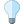#### Versions

You are viewing the RapidMiner Studio documentation for version 9.6 - Check here for latest version

#Quadratic Discriminant Analysis (RapidMiner Studio Core)

## Synopsis

This operator performs quadratic discriminant analysis (QDA) for nominal labels and numerical attributes. Discriminant analysis is used to determine which variables discriminate between two or more naturally occurring groups, it may have a descriptive or a predictive objective.

## Description

This operator performs a quadratic discriminant analysis (QDA). QDA is closely related to linear discriminant analysis (LDA), where it is assumed that the measurements are normally distributed. Unlike LDA however, in QDA there is no assumption that the covariance of each of the classes is identical. To estimate the parameters required in quadratic discrimination more computation and data is required than in the case of linear discrimination. If there is not a great difference in the group covariance matrices, then the latter will perform as well as quadratic discrimination. Quadratic Discrimination is the general form of Bayesian discrimination.

Discriminant analysis is used to determine which variables discriminate between two or more naturally occurring groups. For example, an educational researcher may want to investigate which variables discriminate between high school graduates who decide (1) to go to college, (2) NOT to go to college. For that purpose the researcher could collect data on numerous variables prior to students' graduation. After graduation, most students will naturally fall into one of the two categories. Discriminant Analysis could then be used to determine which variable(s) are the best predictors of students' subsequent educational choice. Computationally, discriminant function analysis is very similar to analysis of variance (ANOVA). For example, suppose the same student graduation scenario. We could have measured students' stated intention to continue on to college one year prior to graduation. If the means for the two groups (those who actually went to college and those who did not) are different, then we can say that the intention to attend college as stated one year prior to graduation allows us to discriminate between those who are and are not college bound (and this information may be used by career counselors to provide the appropriate guidance to the respective students). The basic idea underlying discriminant analysis is to determine whether groups differ with regard to the mean of a variable, and then to use that variable to predict group membership (e.g. of new cases).

Discriminant Analysis may be used for two objectives: either we want to assess the adequacy of classification, given the group memberships of the objects under study; or we wish to assign objects to one of a number of (known) groups of objects. Discriminant Analysis may thus have a descriptive or a predictive objective. In both cases, some group assignments must be known before carrying out the Discriminant Analysis. Such group assignments, or labeling, may be arrived at in any way. Hence Discriminant Analysis can be employed as a useful complement to Cluster Analysis (in order to judge the results of the latter) or Principal Components Analysis.

## Differentiation

###Linear Discriminant Analysis

The QDA performs a quadratic discriminant analysis (QDA). QDA is closely related to linear discriminant analysis (LDA), where it is assumed that the measurements are normally distributed. Unlike LDA however, in QDA there is no assumption that the covariance of each of the classes is identical.

###Regularized Discriminant Analysis

The RDA regularized discriminant analysis (RDA) which is a generalization of the LDA and QDA. Both algorithms are special cases of this algorithm. If the alpha parameter is set to 1, RDA operator performs LDA. Similarly if the alpha parameter is set to 0, RDA operator performs QDA.

## Input

•training set (IOObject)

This input port expects an ExampleSet. It is the output of the Retrieve operator in the attached Example Process. The output of other operators can also be used as input.

## Output

•model (Model)

The Discriminant Analysis is performed and the resultant model is delivered from this output port

•example set (IOObject)

The ExampleSet that was given as input is passed without changing to the output through this port. This is usually used to reuse the same ExampleSet in further operators or to view the ExampleSet in the Results Workspace.

## Parameters

• approximate_covariance_inverseThis parameter indicates whether the inverse of the covariance matrices should be approximated if the actual inverse does not exist. This is activated by default. Range: boolean

## Tutorial Processes

### Introduction to the QDA operator

The 'Sonar' data set is loaded using the Retrieve operator. A breakpoint is inserted here so that you can have a look at this ExampleSet. The Quadratic Discriminant Analysis operator is applied on this ExampleSet. The Quadratic Discriminant Analysis operator performs the discriminant analysis and the resultant model can be seen in the Results Workspace.